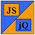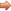# JavaScript & jQuery Tutorials

JS HomeJS IntermediateFunctions

## Functions

Javascript functions are small subprograms which can be called by external code, or recursively by the function itself. This lesson is all about functions, which consist of a function body, can accept parameters and can also return a value to the calling code.

Functions are created using the global object constructor `Function`, via declaration using the `function` statement, or by function expression using the `function` special operator.

All functions are `Function` objects underneath the covers, but as mentioned above there are different ways to set up the function creation. We will look at each way of creating functions as we work through the lesson and also give the pros and cons of using a certain method of function creation.

Values are passed back to the calling code using the `return` statement. If no `return` statement is passed back from a function, the function `undefined`.

## Function Creation Using The `Function` Constructor

The `Function` constructor allows us to create a `Function` object.

`Function` objects created this way are less efficient as they are parsed on function creation as opposed to being parsed with the rest of The JavaScript code.

Below is an example of using the `Function` constructor.

``````
*/
/ Create a Function that takes an argument and
/ returns the square of the argument.
/*
var newFunction = new Function('a', 'return a * a');

``````

Press the button below to action the above code:

## Function Creation Using The `function` Statement

The `function` statement allows us to create a function declaration using a statement.

`Function` objects created this way are parsed with the rest of The JavaScript code and so are more efficient than functions created using the constructor creation method.

Below is an example of using the `function` statement.

``````
*/
/ Create a function statement that takes an argument and
/ returns the square of the argument.
/*
function squareNumber(a) {
return a * a;
}

``````

Press the button below to action the above code:

## Function Creation Using The `function` Special Operator

The `function` special operator allows us to create a `Function` object using an expression. The function expression can have a name or be an anonymous function.

`Function` objects created this way are parsed with the rest of The JavaScript code and so are more efficient than functions created using the constructor creation method.

Below are examples of using the `function` special operator.

``````
*/
/ Create a function operator that takes an argument and
/ returns the square of the argument.
/*
var squareResult = function(a) {
return a * a;
};
alert(squareResult(7) + ' Anonymous function expression');
alert(squareResult(15) + ' Anonymous function expression');

*/
/ Create a named function expression that takes an argument and
/ returns the square of the argument.
/*
var squareResult2 = function getSquare(a) {
return a * a;
};
alert(squareResult2(6) + ' Named function expression getSquare{}');
alert(getSquare(17) + ' Named function expression getSquare{}');

``````

Press the button below to action the above code:

## Function Chaining

The `Function` object also has a couple of handy methods that allow us to use some prototyping of another function as part of the current function. The first method is the `apply()` method which calls a function with a given `this` value and arguments provided as an array. The second method is the `call()` method which calls a function with a given `this` value and arguments provided individually. Apart from this the methods are the same, so we will look at the `apply()` method here.

Below is an example of using the `apply()` method for chaining.

``````
function Country(name, capital) {
this.name = name;
this.capital = capital;
return this;
}
function County(name, capital, county) {
Country.apply(this, arguments);
this.county = county;
}
County.prototype = new Country();
// Define custom override function for County toString().
County.prototype.toString = function CountyToString() {
var county = this.name + ' ' + this.capital + ' ' + this.county;
return county;
}
var essex = new County('England', 'London', 'Essex');

``````

Press the button below to action the above code:

## Lesson 8 Complete

In this lesson we looked at functions and the various ways to create them.

## Related Tutorials

JavaScript Advanced Tutorials - Lesson 8 - Function Recursion & Closures

## What's Next?

In the final lesson of these intermediate tutorials we look at regular expressions